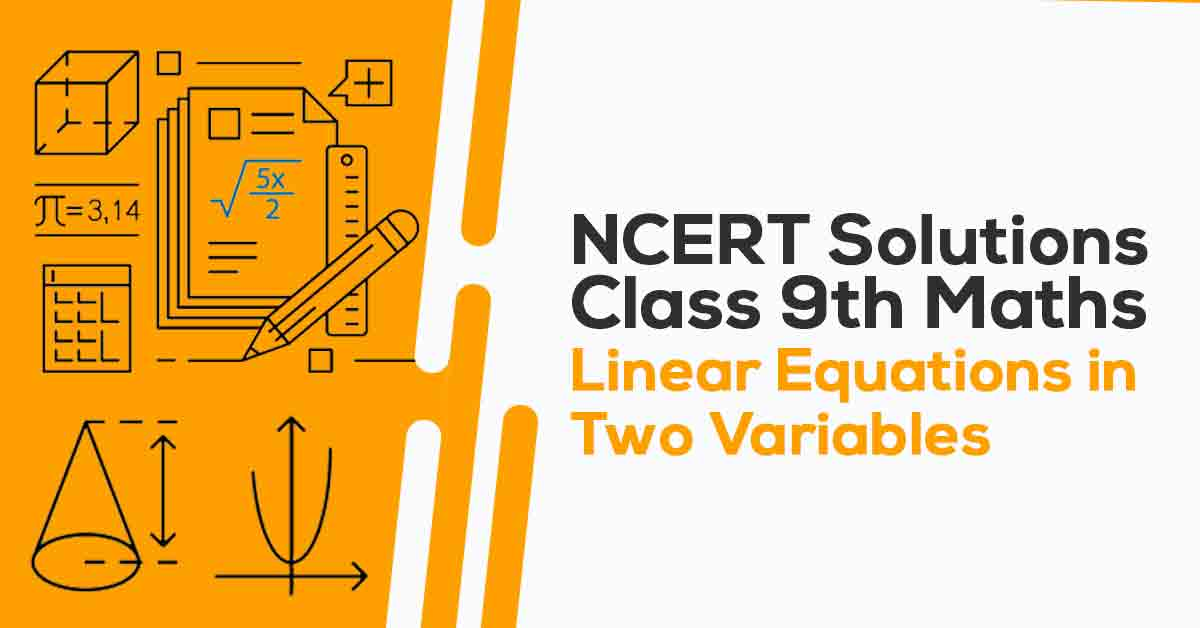Class 9th Math Liner Equations is Two Variables NCERT Solution CBSE 2023

# NCERT Solutions For Class 9 Maths Chapter 4

## NCERT Solutions For Maths Class 9 Chapter 4 Linear Equations in Two Variables - PDF DownloadStudents require NCERT Solutions for Class 9 Maths Chapter 4 Linear Equations in Two Variables for better exam preparation. In this chapter, we will learn that an equation of the form ax + by + c = 0, where a, b, and c are real numbers, such that a and b are not both zero, is called a linear equation in two variables.  A linear equation in two variables has infinitely many solutions. The graph of every linear equation in two variables is a straight line.

These CBSE NCERT Solutions for Class 9 Maths Chapter 4 Linear Equations in Two Variables will help you to practice all the topics in the chapter with ease and score maximum marks in the examination.

What is Linear Equations?

Linear Equation is defined as the condition between two factors that gives a straight line when plotted on a graph.